• matlab画图
2021-11-19 17:54:20

设置白色背景

matlab命令框里面输入  set(0,'defaultfigurecolor','w')

背景变白了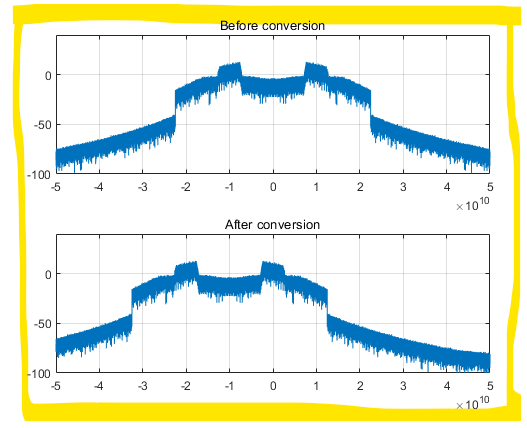曲线、点的操作，坐标轴操作，文字说明

Matlab--用代码让图片更好看_今夜无眠的博客-CSDN博客_matlab修图

Matlab plot画图坐标字体、字号、范围等设置 - 楚千羽 - 博客园

Matlab plot画图 坐标字体、字号、范围、间隔等的设置_Lucky_Xue的博客-CSDN博客_matlab设置坐标轴字体的大小

创建双y轴

创建包含双 y 轴的图。- MATLAB & Simulink- MathWorks 中国

text文本说明

向数据点添加文本说明 - MATLAB text- MathWorks 中国

legend文本说明

plot(rand(4))lgd = legend('One','Two','Three','Four');lgd.FontSize = 14;

legend({'One','Two','Three','Four'},'FontSize',14)

设置图形外边框线宽

set(gca,'linewidth',1.5)

设置网格虚线

grid on;set(gca,'GridLineStyle',':','GridColor','k','GridAlpha',1);

matlab绘图中坐标轴标注设置及图片大小等的设置及输出_apple-mapping的博客-CSDN博客_matlabplot坐标轴标注html css
更多相关内容
• Matlab 使图像填充整个figure窗口，去掉周围白边并保存图像。matlab保存figure中的图片去掉白边。plot和imshow图窗怎么保存图片。
• matlab画图橙色代码使用均匀量化器模拟差分脉冲编码调制（DPCM） 1.简介 DPCM（差分脉冲编码调制）可以看作是Delta编码的概括，其中量化并发送到接收器的信号是当前样本（时间n）与线性预测之间的差。 也就是说，在...
• Matlab画图时-Marker过多的解决方法.pdf
• matlab画图 横坐标为时间轴 非常适合处理时间非常多的情况，也非常适合处理时间非连续的情况。 代码详细，而且注释也很详细。
• matlab图中图工具。即在图中圈定一块，然后用一个放大图显示。 使用方法： 将该文件夹加入matlab的路径中， 使用时先figure一张图 然后在命令行中输入mmzoom 用鼠标在图中圈定一块，出现一个放大的子图 在命令行中...
• matlab画图技巧，颗快速掌握matlab画图常用技巧
• 超全MATLAB绘图索引（含代码）,matlab画图代码,matlab源码.zip
• ## MATLAB画图

千次阅读 2020-02-06 17:32:43
有不少研究者都采用MATLAB进行绘图，因为MATLAB画图功能齐全又不失美观，接下来我将结合具体代码记录一下MATLAB画图的一些操作和心得。 第一段代码： %Fig1. close all clear syms w p c t d pe b we a c=1; d=1; b...

有不少研究者都采用MATLAB进行绘图，因为MATLAB画图功能齐全又不失美观，接下来我将结合具体代码记录一下MATLAB画图的一些操作和心得。

### 第一段代码：

%Fig1.
close all
clear
syms w p c t d pe b we a
c=1; d=1; b=1;
a1 = (4*d*(512*c*d^3*t - b^3*c*t^4 + 8*b^3*c*t^4*(d/(8*(4*d - b*t)))^(1/2) - 320*b*c*d^2*t^2 + 36*b^2*c*d*t^3 + 512*b*c*d^2*t^2*(d/(8*(4*d - b*t)))^(1/2) - 160*b^2*c*d*t^3*(d/(8*(4*d - b*t)))^(1/2)))/(- b^3*t^3 + 36*b^2*d*t^2 - 384*b*d^2*t + 512*d^3);%>0
a0 = 4*c*d*t;
f1 = ezplot(a1,[0 2]);
axis([0 4 0 90])
hold on
f1 = ezplot(a0,[0 4]);
axis([0 4 0 90])
hold on
legend('\it a_1','\it a_0')
xlabel(['\fontsize{7.5} \it t'])
ylabel(['\fontsize{7.5} \it a'])
set(gca,'FontName','Times New Roman','FontSize',7.5)
title(' ')
set(findobj('Type','line'),'color','k')
set(findobj('Type','line'),'LineWidth',2)


首先如果不注意设置参数和图片大小比例的话，MATLAB也会画出很丑的图，如上面的代码跑出来的图片如下：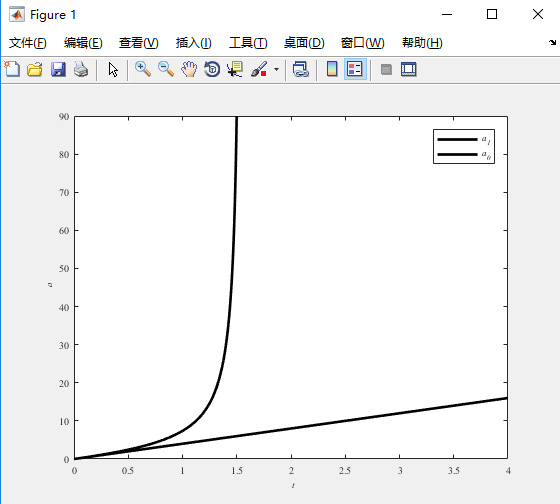可以看到，图片的坐标的标注字体很小，字体类型不好看，图例很小，还有其他各种缺点，使得整张看起来很空旷，不紧凑，美观不足。这样的图片放在论文中，怕是初审在编辑那一关就把你枪毙了。因此，接下来我将对代码进行改善，对图片进行编辑，得到一张更好看的，适合放在文章中的图片。

### 第二段代码：

%Fig1.
close all
clear
syms w p c t d pe b we a
c=1; d=1; b=1; % 控制这些变量为定值，以便下面两个表达式只有两个变量，可以画出二维图
% a1 = @(t)(4*d.*(512*c*d^3.*t - b^3*c.*t.^4 + 8*b^3*c.*t.^4.*(d./(8*(4*d - b.*t))).^(1/2) - 320*b*c*d^2.*t.^2 + 36*b^2*c*d.*t.^3 + 512*b*c*d^2.*t.^2.*(d./(8*(4*d - b.*t))).^(1/2) - 160*b^2*c*d.*t.^3.*(d./(8*(4*d - b.*t))).^(1/2)))./(- b^3.*t.^3 + 36*b^2*d.*t.^2 - 384*b*d^2.*t + 512*d^3);%>0
% a0 = @(t)4*c*d.*t;
a1 = (4*d*(512*c*d^3*t - b^3*c*t^4 + 8*b^3*c*t^4*(d/(8*(4*d - b*t)))^(1/2) - 320*b*c*d^2*t^2 + 36*b^2*c*d*t^3 + 512*b*c*d^2*t^2*(d/(8*(4*d - b*t)))^(1/2) - 160*b^2*c*d*t^3*(d/(8*(4*d - b*t)))^(1/2)))/(- b^3*t^3 + 36*b^2*d*t^2 - 384*b*d^2*t + 512*d^3);%>0
a0 = 4*c*d*t;
figure(1)
f1 = ezplot(a1,[0 2]);  % 画隐函数，函数用字符串表示
set(f1, 'LineStyle', '-', 'LineWidth', 3, 'color', 'k')
axis([0 4 0 90])
hold on
f2 = ezplot(a0,[0 4]);
set(f2, 'LineStyle', '--', 'LineWidth', 3, 'color', 'k')
axis([0 4 0 90])
% gcf是获取图片对象（figure），gca是获取图片内的对象（axe）
% 'XTick'和'YTick'是在指定位置显示刻度，'position'内的四个参数，前两个是图片内对象起点坐标，后两个是图片内对象的宽和高
set(gca,'XTick', 0:1:4, 'YTick', 0:20:80, 'position', [0.15, 0.15, 0.8, 0.7])
% 'XTickLabel'和'YTickLabel'是显示刻度的具体字符
set(gca,'XTickLabel',{'0','1','2','3','4'}, 'YTickLabel', {'0','20','40','60','80'})
set(gca, 'FontSize', 14, 'Fontname', 'Times New Roman', 'FontWeight', 'bold') % 对刻度上的字符字体设置
set(gcf,'position', [150, 100, 500, 500]) % 'position'内的四个参数，前两个是图片起点坐标，后两个是图片的宽和高
[le, lg, ~, ~] = legend( {'a_1', 'a_0'}, 'FontName', 'Times New Roman', 'FontAngle', 'italic'); % 设置图例和它的字体， 并获得两个对象
set(le, 'Box', 'off')
p = get(le,'Position'); % 获得图例的坐标和宽高
p1 = lg(1).Position; % 获得图例内第一个函数图像的坐标
p2 = lg(2).Position; % 获得图例内第二个函数图像的坐标
lg(1).Position = [p1(1)-0.1 p1(2) 0.2]; % 设置图例内第一个函数图像的坐标
lg(2).Position = [p2(1)-0.1 p2(2) 0.2]; % 设置图例内第二个函数图像的坐标
lg(3).XData = [0.1 0.5]; % 设置图例内第一个函数图像，第一个参数表示图像距离左边框的距离，第二个表示图像线的长度
lg(5).XData = [0.1 0.5]; % 设置图例内第二个函数图像，第一个参数表示图像距离左边框的距离，第二个表示图像线的长度
xlabel('t', 'FontName', 'Times New Roman', 'FontSize', 14, 'FontWeight', 'bold', 'FontAngle', 'italic')
ylabel('a', 'FontName', 'Times New Roman', 'FontSize', 14, 'FontWeight', 'bold', 'FontAngle', 'italic')
title(' ')


### 呈现效果：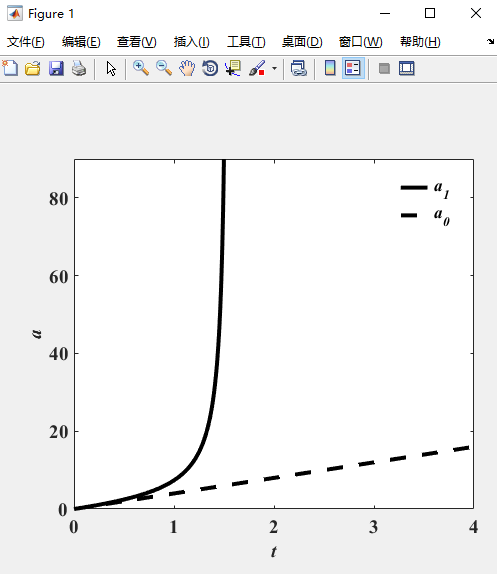利用MATLAB自带的图片工具对上面的图片进行调整，使它更好看。点击上方“查看”–>“属性编辑器”，进入如下页面：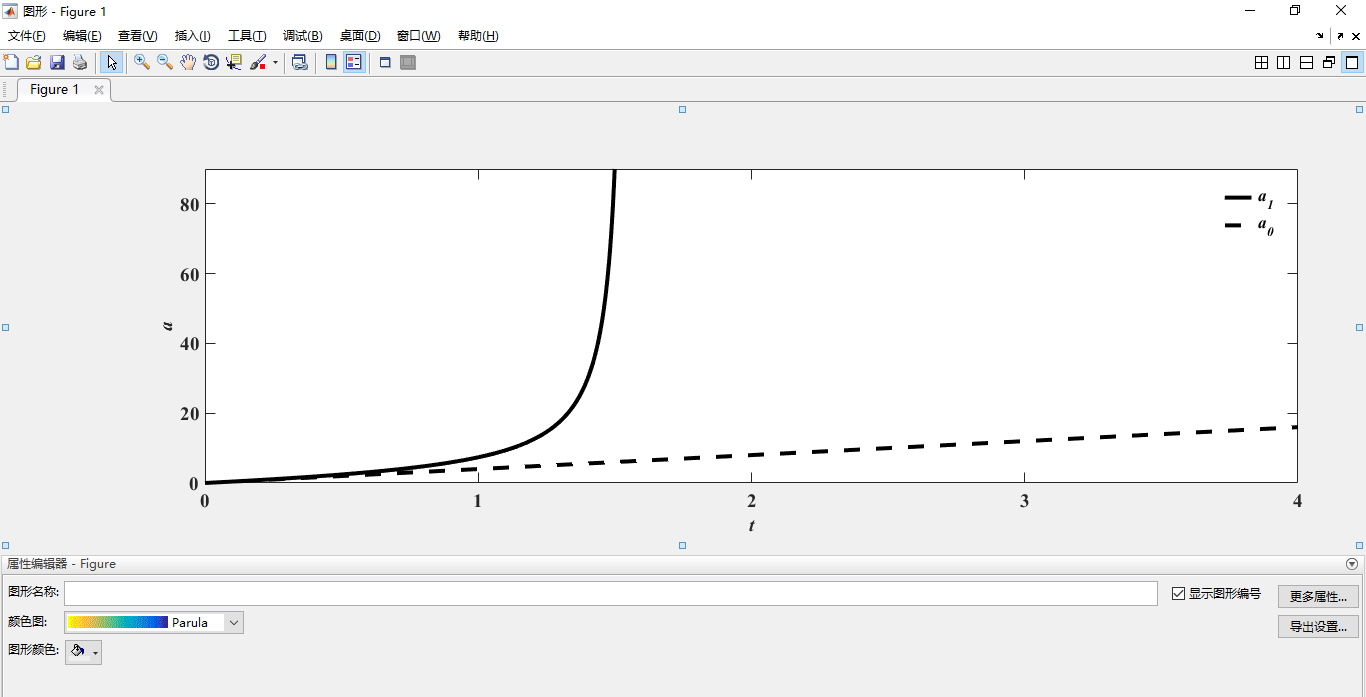点击上方“插入”–>“文本框”，然后输入想附上的文本，我这里输入了“I: 3MR”和“II: 3R”，并且调节边框为无色，设置字体大小为16，加粗，新罗马体，得到下方的页面：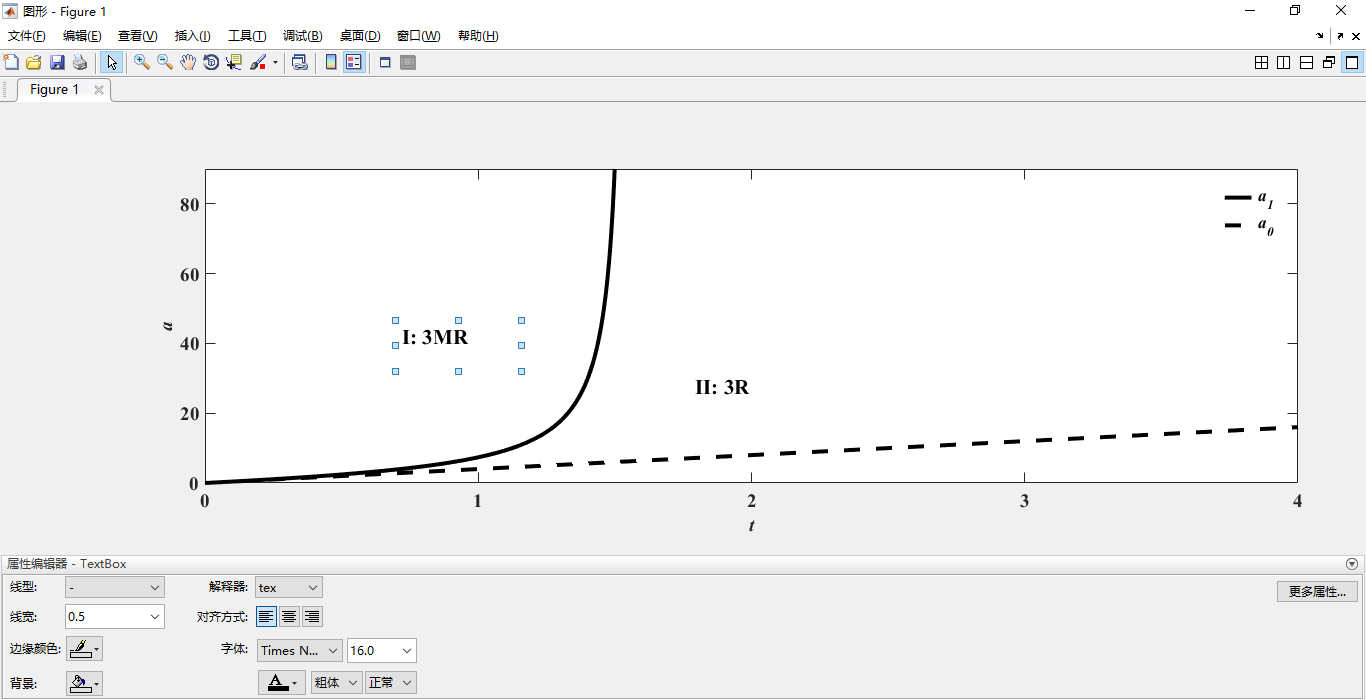点击右上角的小箭头“取消停靠figure 1”，得到如下页面：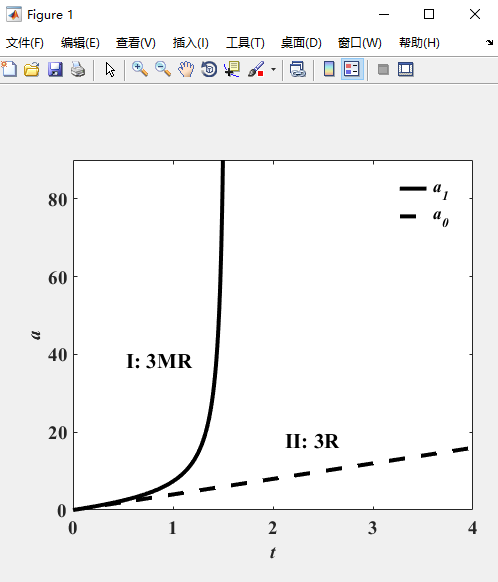点击上方“工具”–>“编辑图形”，拉伸图例，得到如下页面：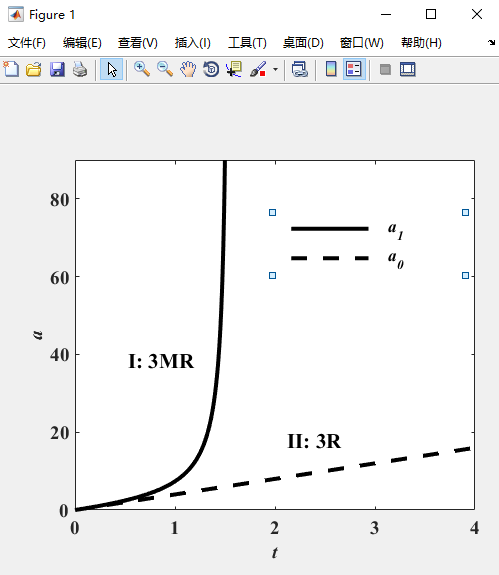点击上方“插入”–>“矩形”，画出矩形将图例套住，然后点击上方“查看”–>“属性编辑器”，将边框加粗，然后“取消停靠figure 1”，得到如下页面：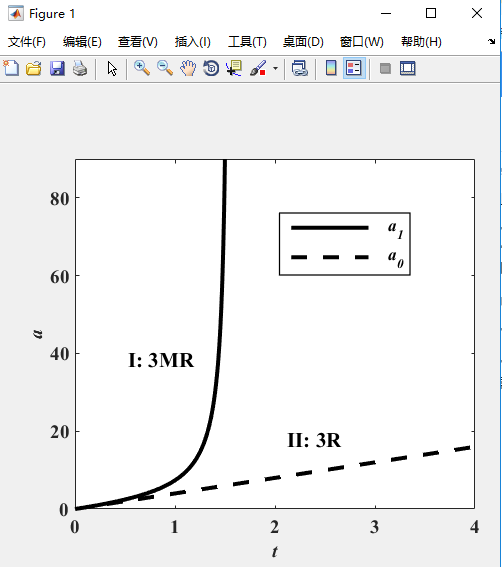最终图片做完。点击上方“文件”–>“另存为”，存成png格式。

### 最终呈现效果：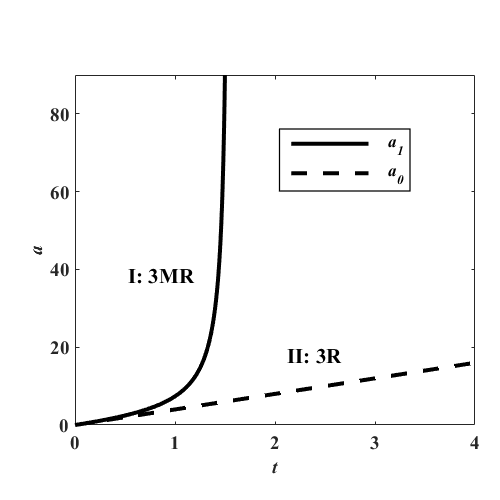可以看到，最终呈现的图片在字体，清晰度，大小，紧凑性各方面都有了很大改进，整体还是很好看的，适合插入论文中。

展开全文• ## MATLAB画图

千次阅读 2021-08-30 17:12:05
matlab画图

目录

图形对象属性

坐标轴

散点图

Line 属性

imagesc

histogram 直方图

subplot

图像保存

其他

# 图形对象属性

set 设置图形对象属性

set(H,Name,Value)：为 H 标识的对象指定其 Name 属性的值

p = plot(1:10);
set(p,'Color','red')% 更改特定线条的颜色


gca 当前坐标区或图
设置当前坐标区的字体大小、刻度方向、刻度长度以及 y 坐标轴范围。

ax = gca; % current axes
ax.FontSize = 12;
ax.TickDir = 'out';
ax.TickLength = [0.02 0.02];
ax.YLim = [-2 2];


figure窗口背景的黑白变换

背景的暂时变化，对于新建的figure无效：
set(gcf,'color','white'); （gcf 返回当前Figure 对象的句柄值）

对于新建的figure同样有效：
colordef black（从白色变为黑色背景）
colordef white（从黑色变白色）
colordef none（变为默认值）

以上修改，你会发现一旦关了Matlab再开启，所有的设置又会变回去，不能永久保存。如果需要永久改变，可以用这个命令：reset(groot)

# 坐标轴

title ：添加标题
在标题中可以包含变量的值，例如：

figure
plot((1:10).^2)
f = 70;
c = (f-32)/1.8;
title(['Temperature is ',num2str(c),' C'])


xlim([xl xr]);ylim([yl yr]);用于限定x轴 y轴上线限制

坐标轴的显示方式
axis off;% 去掉坐标轴
axis tight;% 紧坐标轴
axis equal;% 等比坐标轴

set(gca,'YDir','reverse') 把y轴反转，Y轴值上面小，下面大

set(gca,'YDir','normal') 回到正常

若要为图形删除边框线，则输入命令：

box off
box on   # 加入框线


# 散点图

scatter(x,y,sz,c)
sz：指定圆大小。要绘制大小相等的圆圈，请将 sz 指定为标量。要绘制大小不等的圆，请将 sz 指定为长度等于 x 和 y 的长度的向量。
c：指定圆颜色。要以相同的颜色绘制所有圆圈，请将 c 指定为颜色名称或 RGB 三元组。要使用不同的颜色，请将 c 指定为向量或由 RGB 三元组组成的三列矩阵。

scatter(___,'filled')
填充圆形。可以将 'filled' 选项与前面语法中的任何输入参数组合一起使用。

# Line 属性

线条

Color - 线条颜色

[0 0 0] （默认黑色） | RGB 三元组 | 十六进制颜色代码 | 'r' | 'g' | 'b' | ...

颜色短名称
'r'
绿'g'
'b'
'y'
'k'
'w'
青蓝色'c'

LineStyle - 线型
'-' （默认） | '--' | ':' | '-.' | 'none'

线型说明
'-'实线
'--'虚线
':'点线
'-.'点划线

LineWidth - 线条宽度
0.5 （默认） | 正值
线宽，指定为以磅为单位的正值，其中 1 磅 = 1/72 英寸。如果该线条具有标记，则线条宽度也会影响标记边。

Marker - 标记符号
'none' （默认） | 'o' | '+' | '*' | '.' | ...

说明
'o'圆圈
'+'加号
'*'星号
'.'
's'方形
'd'菱形
'p'五角星
'h'六角星

MarkerIndices - 要显示标记的数据点的索引
1:length(YData) （默认） | 正整数向量 | 正整数标量
要显示标记的数据点的索引，指定为正整数向量。如果不指定索引，MATLAB 将在每个数据点显示一个标记。

 plot(x,y,'-o','MarkerIndices',[1 5 10])
% 在第一、第五和第十个数据点处显示圆形标记。
plot(x,y,'-x','MarkerIndices',1:3:length(y))
% 每隔三个数据点显示一个交叉标记。
plot(x,y,'Marker','square','MarkerIndices',5) % 在第五个数据点显示一个正方形标记。


plot 二维线图

plot(X1,Y1,LineSpec1,...,Xn,Yn,LineSpecn) 设置每个线条的线型、标记符号和颜色。您可以混用 X、Y、LineSpec 三元组和 X、Y 对组。指定线型、颜色和标记：

plot(x,y1,'g',x,y2,'b--o',x,y3,'c*')


plot(ax,___)
将在由 ax 指定的坐标区中，而不是在当前坐标区 (gca) 中创建线条。选项 ax 可以位于前面的语法中的任何输入参数组合之前。

ax1 = subplot(2,1,1); % top subplot
x = linspace(0,3);
y1 = sin(5*x);
plot(ax1,x,y1)
title(ax1,'Top Subplot')
ylabel(ax1,'sin(5x)')

ax2 = subplot(2,1,2); % bottom subplot
y2 = sin(15*x);
plot(ax2,x,y2)
title(ax2,'Bottom Subplot')
ylabel(ax2,'sin(15x)')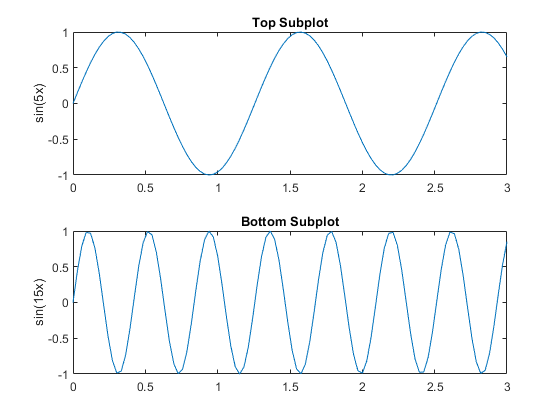# imagesc

https://www.mathworks.com/help/matlab/ref/imagesc.html#d122e631230

• imagesc(A) 将矩阵A中的元素数值按大小转化为不同颜色，并在坐标轴对应位置处以这种颜色染色
• imagesc(x,y,A) x,y决定坐标范围，x,y应是两个二维向量，即x=[x1 x2],y=[y1 y2],matlab会在[x1,x2]*[y1,,y2]的范围内染色。
imagesc(___,clims)


clims指定映射到colormap的第一个和最后一个元素的数据值
clims=[cmin-cmax]
其中小于或等于tocminmap的值映射到colormap中的第一种颜色，大于或等于tocmaxmap的值映射到colormap中的最后一种颜色。

# histogram 直方图

histogram(X) 基于 X 创建直方图。
histogram 函数使用自动 bin 划分算法，然后返回均匀宽度的 bin，这些 bin 可涵盖 X 中的元素范围并显示分布的基本形状。
histogram 将 bin 显示为矩形，这样每个矩形的高度就表示 bin 中的元素数量。
例如：生成 10,000 个随机数并创建直方图。histogram 函数自动选择合适的 bin 数量，以便涵盖 x 中的值范围并显示基本分布的形状。

x = randn(10000,1);
h = histogram(x)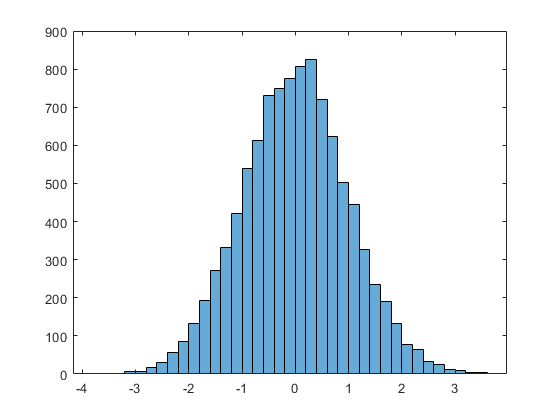histogram(X,nbins) 使用标量 nbins 指定的 bin 数量。
例如：对分类为 25 个等距 bin 的 1,000 个随机数绘制直方图：

x = randn(1000,1);
nbins = 25;
h = histogram(x,nbins)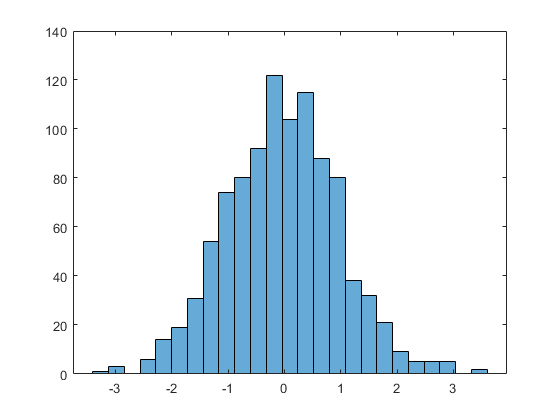指定 histogram 函数的输出参数时，它返回一个二元直方图对象。可以使用该对象检查直方图的属性:Histogram 属性

counts = h.Values
counts = 1×25
..........


调整直方图属性
通过显式设置 bin 数按精细颗粒级别调整 bin。
h.NumBins = 31;
h.BinEdges = [-3:3];
通过向量指定 bin 边界。向量中的第一个值是第一个 bin 的左边界。最后一个值是最后一个 bin 的右边界。
h.EdgeColor = 'r';

指定直方图的 bin 边界

x = randn(1000,1);
edges = [-10 -2:0.25:2 10];
h = histogram(x,edges);


生成 1,000 个随机数并创建直方图。将 bin 边界指定为向量，使宽 bin 在直方图的两边，以捕获不满足|x|<2 的离群值。第一个向量元素是第一个 bin 的左边界，而最后一个向量元素是最后一个 bin 的右边界。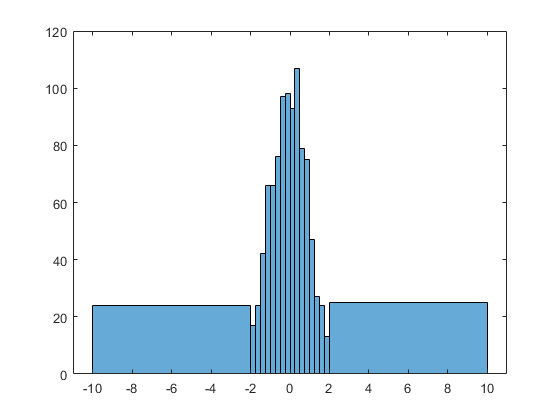histogram(___,Name,Value) 使用前面的任何语法指定具有一个或多个 Name,Value 对组参数的其他选项。例如:
可以指定 'BinWidth' 和一个标量以调整 bin 的宽度。
或指定 'Normalization' 和一个有效选项（'count'、'probability'、'countdensity'、'pdf'、'cumcount' 或 'cdf'）以使用不同类型的归一化

histogram(C)（其中 C 为分类数组）通过为 C 中的每个类别绘制一个条形来绘制直方图。
histogram(C,Categories) 仅绘制 Categories 指定的类别的子集。

A = [0 0 1 1 1 0 0 0 0 NaN NaN 1 0 0 0 1 0 1 0 1 0 0 0 1 1 1 1];
C = categorical(A,[1 0 NaN],{'yes','no','undecided'})
h = histogram(C,'BarWidth',0.5)
% 使用相对条形宽度 0.5 绘制投票的分类直方图。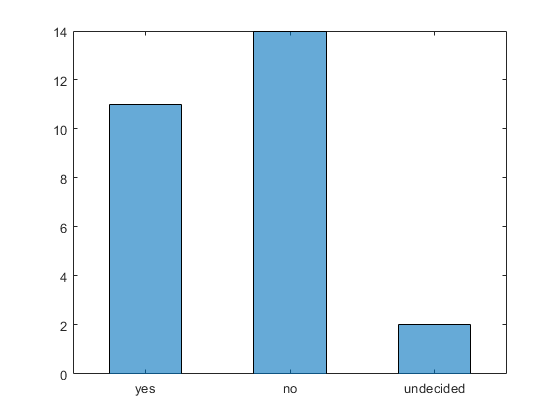hist（）

hist有直方图的意思，直方图也被称为频数直方图，它用来显示数据集的分布情况。

hist(x) 基于向量 x 中的元素创建直方图条形图。x 中的元素有序划分入 x 轴上介于 x 的最小值和最大值间的 10 个等间距 bin 中。hist 将 bin 显示为矩形，这样每个矩形的高度就表示 bin 中的元素数量。

[counts,centers] = hist(___) 返回一个附加行向量 centers，指示 x 轴上每个 bin 中心的位置。
例如：生成 1,000 个随机数。计算 10 个等间距 bin 的每一个中有多少个数字。返回 bin 计数和 bin 中心。

x = randn(1000,1);
[counts,centers] = hist(x)


counts = 1×10

4 27 88 190 270 243 123 38 13 4

centers = 1×10

-2.8915 -2.2105 -1.5294 -0.8484 -0.1673 0.5137 1.1947 1.8758 2.5568 3.2379
使用 bar 绘制直方图。

bar(centers,counts)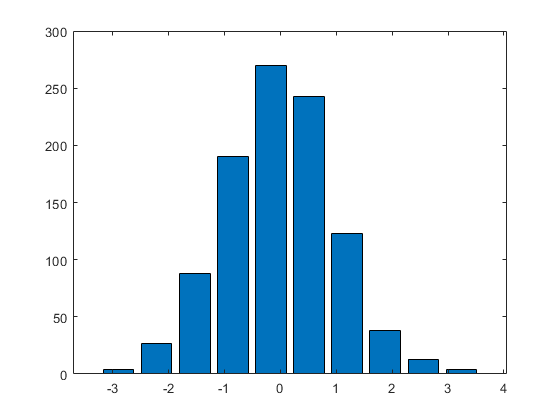# subplot

subplot(m,n,p) 将当前图窗划分为 m×n 网格，并在 p 指定的位置创建坐标区。

但用这个方法画出来的图周围会有很大的白边，如果要去除它需要对每一个子图设置准确位置：

subplot('position',[0.05 0.71 0.9 0.25])

# 图像保存

1、使用imwrite函数

imwrite(img,'result.jpg');
这种方法保存图像大小和显示的大小是一样的。
注意：用imwrite保存的图片只有图片本身，没有其他内容。

将plot/imagesc产生的图片使用imwrite()保存到本地

x = -10:0.1:10; % x赋值
y = x.^2; % y赋值
fig = figure; % 新建一个figure，并将图像句柄保存到fig
plot(x,y,'.') % 用"."的形式将x，y表现在上面生成的图像中
legend({'y=x^2'},'Location','northwest') % 在图像的左上角生成图例
frame = getframe(fig); % 获取frame
img = frame2im(frame); % 将frame变换成imwrite函数可以识别的格式
imwrite(img,'a.png'); % 保存到工作目录下，名字为"a.png"



getframe捕获坐标区或图窗作为影片帧

F = getframe 捕获显示在屏幕上的当前坐标区作为影片帧。
F 是一个包含图像数据的结构体。getframe 按照屏幕上显示的大小捕获这些坐标区它并不捕获坐标区轮廓外部的刻度标签或其他内容。

F = getframe(ax) 捕获 ax 标识的坐标区而非当前坐标区。
F 是一个结构体，其中包含的 cdata 字段中包含捕获的图像数据。
使用 imshow 显示捕获的图像数据

plot(rand(5))
F = getframe;

figure
imshow(F.cdata)
imwrite(frame.cdata，path) %保存图像


F = getframe(fig) 捕获由 fig 标识的图窗。如果您需要捕获图窗窗口的整个内部区域（包括坐标区标题、标签和刻度线），则指定一个图窗。捕获的影片帧不包括图窗菜单和工具栏。

2、saveas命令
saveas(gca, filename, fileformat)，其中的三个参数：
（1）gca：图形句柄，如果图形窗口标题栏是“Figure 3”,则句柄就是3；也可以直接用gcf获取当前窗口句柄。
（2）filename：单引号字符串，指定文件名
（3）fileformat：单引号字符串，指定存储格式

saveas(gcf,'save.jpg'); %保存当前窗口的图像
saveas(2,'save.jpg'); %保存Figure 2窗口的图像
saveas(gcf,'C:\Users\jin\Desktop\sinx.jpg')
% 其中C:\Users\jin\Desktop\是想要保存图形的路径。


saveas保存的结果，有白边。如果想将生成的图片用于后续的图像处理，建议使用imwrite函数，否则，用哪种保存函数都可以。

3、print

打印图窗或保存为特定文件格式

print('-dpng','-r1000','20210317-1902.png')

指定分辨率，将图窗另存为图像文件。【产生位图（质量好坏与分辨率有关），适用于伪彩图。MATLAB直接复制图窗得到矢量图，适用于曲线图】

保存图片并批量命名时，冒号：不能作为命名。

保存自定义的颜色图 color bar

save(filename,variables)

% 保存设置的颜色图
ax = gca;
MyColormap = colormap(ax);
save('MPLNETmap','MyColormap')


# 其他

图中曲线按顺序画出：

pause(a)表示程序暂停a秒后继续执行

当程序中只有pause，并没有参数a，按任意键程序才继续执行。可用于hold on前后。

MATLAB气象绘图汇总
https://mp.weixin.qq.com/s/6KxZmA-p1ORaKEmA8mno5g

展开全文• C#调用matlab画图，将图像嵌入Winform窗体，并解决首次画图慢的问题 预览和介绍请看：http://blog.csdn.net/yxy244/article/details/79305757
• 利用c#调用matlab画图，特别是对于三维图等对图形要求较高的场合。c#
• matlab画图时图例的设置方法的详细介绍，里面有示例代码
• 西安建筑科技大学 信控学院 第5章 MATLAB绘图 5.1 二维图形 5.2 三维图形 5.3 三维图形的精细处理 5.4 隐函数绘图 5.5 低层绘图操作(选讲) 5.6 光照和材质处理 5.7 图像显示与动画制作 5.1 二维图形 5.1.1 绘制二维...
• MATLAB画图——基础篇 在MATLAB使用的过程中，学会画图是一项必要的技能。在这里，我总结了部分简单的画图函数，同时附上代码（本文中的程序为了方便给出的数据都很简单，大家可以自己去尝试其他数据）。这对刚刚...

MATLAB画图——基础篇

在MATLAB使用的过程中，学会画图是一项必要的技能。在这里，我总结了部分简单的画图函数，同时附上代码（本文中的程序为了方便给出的数据都很简单，大家可以自己去尝试其他数据）。这对刚刚开始接触MATLAB的小白来说，我认为还是很有帮助的。

# 一、plot（）函数

## 1.二维图形

### （1）绘图选项

线型颜色标记符号
-实线b蓝色.点s方块
：虚线g绿色o圆圈d菱形
.-点划线r红色x叉v朝下三角符号
-双划线c青色+加号^朝上三角符号
m品红*星号<朝左三角符号
y黄色>朝右三角符号p五角星
k黑色h六角星
w白色

### （2）图形的辅助标注和窗口的分割

title（图形说明）
xlabel（x轴说明）
ylabel（y轴说明）
text（x，y图形说明）——在x，y轴处添加文字说明
legend（图例一，图例二，…）
subplot（m，n，p）——将绘图区域分割成m*n个子区域，并按照行从左至 右，从上至下依次编号。p表示第p个绘图子区域。

注意：如果是要两个图画到同一个坐标里面，则在两个plot函数之间添加一行hold on

### （3）格式

plot（x）——缺省自变量绘图格式
plot（x，y）——基本格式。以y（x）的函数关系作图。如果y是n*m的矩 阵，则x为自变量，作出m条曲线。
plot（x1，y1，x2，y2，…，xn，yn）——多条曲线绘图格式
plot（x1，y1，选项1，x2，y2，选项2，…，xn，yn，选项n）——含选项的绘图格式x1=[1 2 3 4 5 6 7 8 9];
x2=[2 4 6 8 10 12 14 16 18];
y1=[1 4 9 16 25 36 49 64 81];
y2=[18 16 14 12 10 8 6 4 2];
subplot(4,1,1);plot(x1);title('例一');xlabel('自变量');ylabel('因变量');
subplot(4,1,2);plot(x1,y1);title('例二');xlabel('自变量');ylabel('因变量');
subplot(4,1,3);plot(x1,y1,x2,y2);title('例三');xlabel('自变量');ylabel('因变量');
subplot(4,1,4);plot(x1,y1,'m+',x2,y2,'c*');title('例四');xlabel('自变量');ylabel('因变量');


## 2.三维图形

### （1）格式

plot3（x1，y1，z1，‘选项一’，x2，y2，z1，‘选项二’，…）
x，y，z是长度相同的向量：一条曲线
x，y，z是维度相同的矩阵：多条曲线

### （2）网格矩阵生成函数：meshgrid

[X,Y]=meshgrid(x,y)
x，y是给定的向量，X,Y是网格划分后得到的网格矩阵
注意，这个函数用来生成网格矩阵，不是直接用来画图的，配合mesh使用。

### （3）mesh函数

mesh(X,Y,Z,C)
X,Y：网格坐标矩阵
Z：网格点上的高度矩阵
C：确定不同高度下的颜色范围

meshc：在mesh基础上增加等高线。
meshz：在mesh基础上增加底座。

### （4）surf函数

surf（X,Y,Z,C）——绘制由矩阵X,Y,Z确定的着色的三维表面图，参数含义同mesh
surfc——与surf相同，在此基础上增加等高线
surfl——与surf相同，在此基础上增加光照效果
注意：使用surfl的时候，C为一个二维或者三维向量。表示光源与曲面之间的方位。

x1=[1 2 3 4 5 6 7 8 9];
x2=[2 4 6 8 10 12 14 16 18];
z1=[1 2 3 4 5 6 7 8 9];
y1=[1 4 9 16 25 36 49 64 81];
y2=[18 16 14 12 10 8 6 4 2];
z2=[1 2 3 4 5 6 7 8 9];
Z=[1 2 3 4 5 6 7 8 9;
2 3 4 5 6 7 8 9 10;
3 4 5 6 7 8 9 10 11;
4 5 6 7 8 9 10 11 12;
5 6 7 8 9 10 11 12 13;
6 7 8 9 10 11 12 13 14;
7 8 9 10 11 12 13 14 15;
8 9 10 11 12 13 14 15 16;
9 10 11 12 13 14 15 16 17];
s=[40;60];
subplot(2,3,1);plot3(x1,y1,z1,x2,y2,z2);
subplot(2,3,2);[X,Y]=meshgrid(x1,y1);mesh(X,Y,Z,Z);
subplot(2,3,3);meshc(X,Y,Z,Z);
subplot(2,3,4);meshz(X,Y,Z,Z);
subplot(2,3,5);surfc(X,Y,Z,Z);
subplot(2,3,6);surfl(X,Y,Z,s);# 二、特殊图形

## 1.对数坐标图

semilogx(x,y)：x轴对数刻度，y轴线性刻度。
semilogy(x,y)：x轴线性刻度，y轴对数刻度。
loglog(x,y)：x，y都是对数刻度。x=[1 2 3 4 5 6 7 8 9];
y=[2 3 4 5 6 7 8 9 10];
subplot(3,1,1);semilogx(x,y);
subplot(3,1,2);semilogy(x,y);
subplot(3,1,3);loglog(x,y);


## 2.极坐标图

polarplot (x,y): 在极坐标中绘制线条
polarscatter (x,y):极坐标中的散点图
polarhistogram (x,y) : 极坐标中的直方图（y为一个整数）
compass (x,y): 绘制从原点发射出的箭头
ezpolar (‘函数’):易用的极坐标绘图函数x=[0.1 0.2 0.3 0.4 0.5 0.6 0.7 0.8 0.9];
y=[0.2 0.3 0.4 0.5 0.6 0.7 0.8 0.9 0.10];
subplot(3,2,1);polarplot(x,y);
subplot(3,2,2);polarscatter(x,y);
subplot(3,2,3);polarhistogram(x,6);
subplot(3,2,4);compass(x,y);
subplot(3,2,5);ezpolar('1+2*sin(x)');


## 3.条形图

bar(y)——若y为向量，则分别显示每个分量的高度。若为矩阵，则把y分解为行向量，在分别画出。
bar(x,y)——在指定的横坐标x上画出y。x为单增向量。
bar(…,width)——设置条形的相对宽度和控制在一组内条形的间距，默认值为0.8。
bar(…,‘style’)——指定条形的排列类型。类型有“group”和“stack”。
group：若y为n*m矩阵，则bar显示n组，每组m个条形图
stack：对矩阵的每一个行向量显示在一个条形中，条形的高度为该行向量中的分量和，其中同一条形中的每个向量用不同的颜色显示出来，从而可以显示每个向量中的分布。

三维格式为bar3x=[0.1 0.2 0.3 0.4];
y=[0.1 1.1 5.4 3.4 2.3 4.5 3.2 3.4 5.6];
c=x+x';
subplot(2,2,1);bar(c);title('例一');
subplot(2,2,2);bar(x,c);title('例二');
subplot(2,2,3);bar(x,c,1);title('例三');
subplot(2,2,4);bar(x,c,'stack');title('例四');


## 4.面积图

area(x)——与plot(x)一样，但是将所得的曲线下方的区域填充颜色。
area(x,y)——y为向量，与plot(x，y)一样，但是将所得的曲线下方的区域填充颜色。
area(x,A)——矩阵A的第一行对向量x绘图，然后依次是下一行所有行值的和对向量x绘图，每个区域有各自的颜色。
area(…,leval)——将填色部分改为由连线图到y=leval的水平之间的部分。x=[0.1 0.2 0.3 0.4];
y=[0.1 1.1 5.4 3.4];
c=x+x';
subplot(2,2,1);area(x);title('例一');
subplot(2,2,2);area(x,y);title('例二');
subplot(2,2,3);area(x,c);title('例三');
subplot(2,2,4);area(x,c,1);title('例四');


## 5.饼状图

pie(x)——用x中的数据画一个饼状图。x中的每一个元素代表饼状图中的一部分。
pie(x,explode)——从饼状图中分离出一部分，explode是与x同维的矩阵。当所有元素为零时，饼状图各部分连一起组成一个圆。当其中有非零元时，元素对应的扇形往外移。

三维格式为pie3x=[0.1 0.2 0.3 0.4];
y=[0 1 0 1];
subplot(2,1,1);pie(x);title('例一');
subplot(2,1,2);pie(x,y);title('例二');


## 6.双y轴

yyaxis left——坐标轴在左边
yyaxis right——坐标轴在右边x=[0.1 0.2 0.3 0.4];
y1=[4 5 6 7];
y2=[1 2 3 4];
yyaxis left;
plot(x,y1);
yyaxis right;
plot(x,y2);
title('例一');


## 7.罗盘图

compass(x,y)——x，y为n维向量
compass(z)——z为n维复数向量
compass(x,y，选项)——选项为箭头的颜色，线型等。x=[0.1 0.2 0.3 0.4];
y=[4 5 6 7];
z=[1+2*i 2+2*i 3+3*i 4+4*i];
subplot(2,2,1);compass(x,y);title('例一');
subplot(2,2,2);compass(z);title('例二');
subplot(2,2,3);compass(x,y,'m*');title('例三');


## 8.羽毛图

feather函数
用法与罗盘图相同x=-pi:pi/10:pi;
y=sin(x);
z=[1+2*i 2+2*i 3+3*i 4+4*i];
subplot(2,2,1);feather(x,y);title('例一');
subplot(2,2,2);feather(z);title('例二');
subplot(2,2,3);feather(x,y,'m*');title('例三');


## 9.矢量图

quiver(u,v)——v为mn矩阵，绘出在范围x=1：n，y=1：m的坐标系中由u和v定义的向量
quiver(x,y,u,v)——x为n维向量，y为m维向量。u和v为m
n维矩阵。则画出由x，y确定的每一个由u和v定义的向量。
quiver(…,scale)——对向量的长度进行处理。x=-pi:pi/10:pi;
y=sin(x);
for i=1:1:21
u(i)=i;
v(i)=i;
end
subplot(2,2,1);quiver(x,y);title('例一');
subplot(2,2,2);quiver(x,y,u,v);title('例二');
subplot(2,2,3);quiver(x,y,u,v,0.5,'m*');title('例三');


## 10.散点图

scatter(x,y)——x，y为向量，且长度相同。
scatter(x,y,sz)——sz标记面积，默认36。
scatter(x,y,sz,c)——c标记颜色。x=-pi:pi/10:pi;
y=sin(x);
for i=1:1:21
u(i)=i;
v(i)=i;
end
subplot(2,2,1);scatter(x,y);title('例一');
subplot(2,2,2);scatter(x,y,10);title('例二');
subplot(2,2,3);scatter(x,y,50,'m*');title('例三');


# 总结

本文参考了很多其他的文章，这也是我第一次写，有写的不好的地方希望大家多提提意见。另外，本文也是当成笔记来写的，因此有一些地方写的很简洁，希望大家多多包涵。

# 参考

展开全文• 还是很不错 GitHub - peijin94/matlabPlotCheatsheet: A cheatsheet for those who plot with MATLAB 上面的图可以作为一个我们快速查看的笔记，详细了解MATLAB画图还不够，接下来带大家来详细盘一盘画图的一些参数 ...
• matlab中，使用plot画图实例，能熟练掌握使用plot进行画图
• 针对多径信道的信道均衡，演示了几种经典的算法。可以直接运行。画图时是动态的，做得很精细。
• 很不错的matlab画图学习教程 大家可以学习下
• mfc 调用matlab 画图，（matlab版本是7.0）.封装成类了 配置方法参考 http://blog.csdn.net/woqunokia/article/details/53927702
• matlab画图aa(:1)表示取aa的第一列，仿真时间aa(:2)表示取aa的第二列，示波器的输入一aa11(:3)表示取aa11的第二列，示波器的输入二--r表示曲线2显示的形式和颜色，这里是（red)设置曲线1的粗细设置曲线2的粗细设置...
• MATLAB画图的ppt-MaTLAB画图.rar 分享：MATLAB画图基础讲义。 第一页介绍： matlab语言的绘图功能 • 不仅能绘制几乎所有的标准图形， 而且其表现形式也是丰富多样的。 • matlab语言不仅具有高层绘图能 ...
• ## matlab画图入门教程

千次阅读 2021-02-01 13:21:47
**matlab画图：**图像是数据结果的一种可视化表现，它能直观的体现你的数据结果，并且能体现你获得结果的准确性，在当前的大数据时代，在做数据分析的时候，将其可视化可以直观多维的展示数据，可以让人们更好的发现......

# matlab画图matlab 订阅